# Two pipes

One pipe fill one-fifth volume 20 minutes before by second one. The two pipes together will fill the tank in two hours. How long is will fill tank each pipe separately?

a =  200 min
b =  300 min

### Step-by-step explanation:

Our quadratic equation calculator calculates it.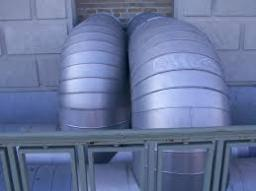Did you find an error or inaccuracy? Feel free to write us. Thank you!Tips to related online calculators
Looking for help with calculating roots of a quadratic equation?
Do you have a linear equation or system of equations and looking for its solution? Or do you have a quadratic equation?
Do you want to convert time units like minutes to seconds?

## Related math problems and questions:

• 2 pipes2 pipes can fill a tank in 35 minutes. The larger pipe alone can fill the tank in 24 minutes less time than the smaller pipe. How long does each pipie take to fill the tank alone?
• Two pipesHow long will the pool be filled with a double supply pipe if it takes the pool to fill the first pipe by 4 hours longer and the second pipe 9 hours longer than both pipes open at the same time?
• Three pumps together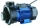One pump fills the tank in 1.5 hours, the second in 2 hours, and the third in 3 hours 20 minutes. How many minutes will the tank fill with three pumps if they work simultaneously?
• Two pumpsPump A takes twice as long to fill a tank as B. Together they take 2 hours to fill the tank. How long does each one take to fill the tank by itself?
• Inlet minus outlet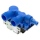Water flows into the tank through two pipes and flows out of the third pipe spontaneously. Pipe A itself would fill the tank in 3 hours, pipe B itself would fill in 4 hours, and pipe C would flow out in 12 hours. How long will the tank be full if all thre
• Water reservoir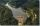The water reservoir is filled through one inlet 4 hours later than both together, then another inlet 9 hours later. For how long is filled by each separately?
• Two pumps togetherThe first pump will fill the tank itself in 3 hours and the second one in 6 hours. How many hours will the tank be full if both pumps are worked at the same time?
• Two workers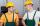Two workers work together and perform the job in 12 hours. If one has done half the work and then the second worker next half of the work it will take 25 hours. How long would it take job them separately?
• Tank 9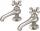The tank with volume V is filled with one pump for three hours and by the second pump for 5 hours. When both pumps run simultaneously, calculate: a) how much of the total volume of the tank is filled in one hour b) for how long is the tank full
• Tractors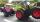Two tractors plow the field in 4 hours together. If the first tractor plow half of the field and then the second tractor completed the job, it would take 9 hours. How many hours does the field plow for each tractor separately?
• Repair pipe20 workers had to repair broken pipes in 30 days. After fourteen days, four laborers joined them. How long did the pipe repair work last?
• Two pumps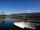The first pump fills the tank in 24 hours second pump in another 40 hours. For how long will it take to fill the tank if it operates both pumps at the same time?
• 4 pipes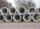The tank flows out by 4 pipes in 6 hours 120 hl water. How much water flows out of 5 pipes of the same diameter in 14 hours?
• Water reservoir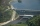One drain hole emptied the water from the tank for 20 hours, with the second drain for 30 hours. How long will the water drain from this tank if we open two drains simultaneously?
• Three tributaries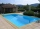It is possible to fill the pool with three tributaries. The first would take 12 hours, the second 15 hours, and the third 20 hours. The day before the summer season began, the manager opened all three tributaries simultaneously. How long did it take to fi
• Tank No 8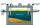Tank is filled by one inlet valve with a flow rate of 12 liters per second in 72 minutes. How long take the tank to fill, if we open half an hour after one more inlet?
• Two workers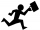One worker makes a part 4 hours and the second 9 hours later than they would make the same part together. How long does it take for each worker to make the part himself?